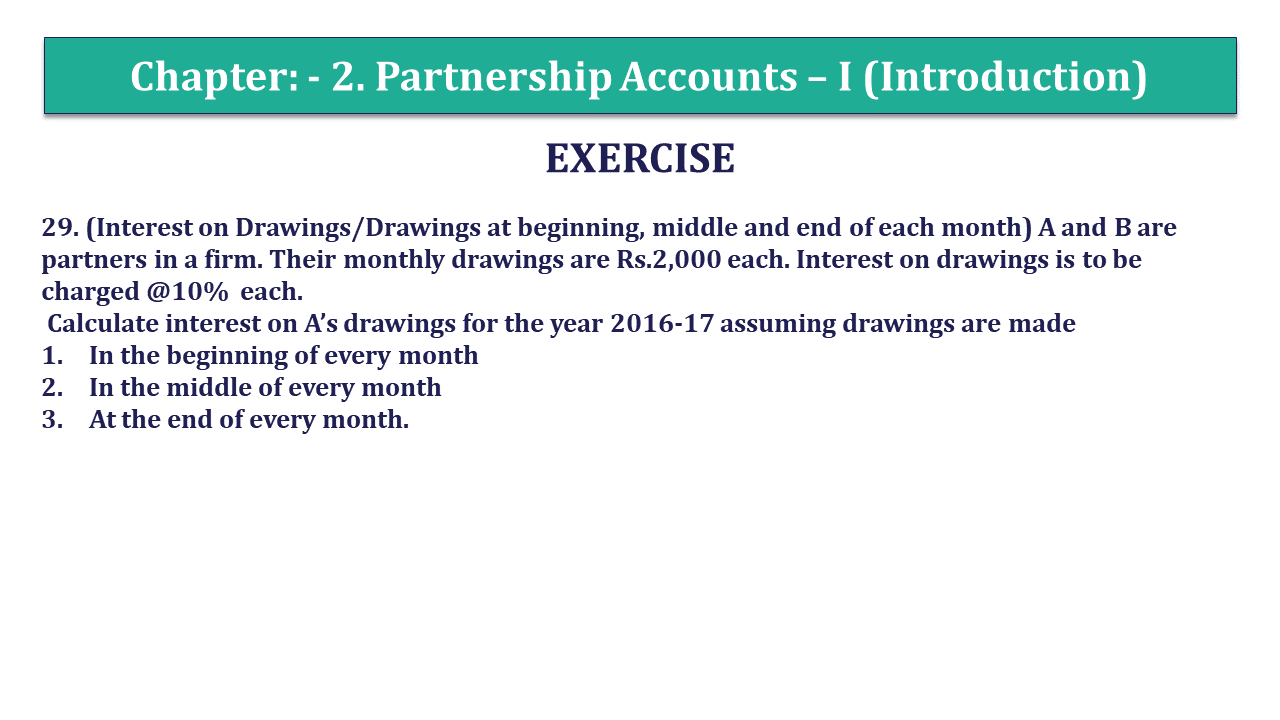# Question 29 Chapter 2 of +2 Part-1 – USHA Publication 12 Class Part – 1Q-29 - CH-2 - Usha +2 Book 2018 - Solution

Question 29 Chapter 2 of +2-Part-1

29. (Interest on Drawings/Drawings at beginning, middle and end of each month) A and B are partners in a firm. Their monthly drawings are Rs.2,000 each. Interest on drawings is to be charged @10% each.
Calculate interest on A’s drawings for the year 2016-17 assuming drawings are made
1.In the beginning of every month
2.In the middle of every month
3. At the end of every month.

### The solution of Question 29 Chapter 2 of +2 Part-1: –

Calculation of interest on partner’s Drawings
If partners withdrawal the same amount of drawing on a regular basis then we can calculate the interest on drawing with help of following formula: –
Interest on Drawing = Total Drawings X Rate of Interest X Period/12 Months
Rate of Interest = 10%
Period = the period/time will be calculated with the help of following formula: –

 Period = Time left after 1st withdrawal + Time left after Last withdrawal 2

1. Calculation of interest on Muthu’s Drawings:

A’s withdrawal at the beginning of every month = 2,000 p.m. Therefore, total drawings of the year= Rs.24,000.
It means 1st withdrawal has made on 01-04-2016
So, Time left from 1-4-2016 to 1-3-17 is 12 Months
And last withdrawal has made on 1-3-2017
So, Time left from 1-3-2017 to 31-3-2017 is 1 month.

Now, put this time period in the formula

 Period = 12 + 1 2
 Period = 13 2
 Period = 6.5

Total Drawings = 24,000

 Interest on Drawing = 24,000 X 10 X 6.5 100 12 Interest on Drawing = 1300/-

2. Drawings in the middle of every month

A’s withdrawal at the middle of every month = 2,000 p.m. Therefore, total drawings of the year= Rs.24,000.
It means 1st withdrawal has made on 15-04-2016
So, Time left from 15-4-2016 to 1-3-17 is 11 months and 15 days. = 11.5 months
And last withdrawal has made on 15-3-2017
So, Time left from 15-3-2017 to 31-3-2017 is 15 days = 0.5 month
Now, put this time period in the formula:

 Period = 11.5 + 0.5 2
 Period = 12 2
 Period = 6

Total Drawings = 24,000

 Interest on Drawing = 24,000 X 6 X 6 100 12 Interest on Drawing = 1200/-

3. Drawings at the end of every month

A’s withdrawal at the end of every month = 2,000 p.m. Therefore, total drawings of the year= Rs.24,000.
It means 1st withdrawal has made on 31-04-2016
So, Time left from 31-4-2016 to 31-3-17 in 11 Months
And last withdrawal has made on 1-3-2017
So, Time left from 31-3-2017 to 31-3-2017 is 0 months.

 Period = 11.5 + 0 2
 Period = 11 2

 Period = 5
 Interest on Drawing = 24,000 X 6 X 5.5 100 12 Interest on Drawing = 1,100/-

Comment if you have any questions.

Also, Check out the solved question of previous Chapters: –